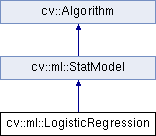OpenCV  3.2.0 Open Source Computer Vision
cv::ml::LogisticRegression Class Referenceabstract

Implements Logistic Regression classifier. More...

#include "ml.hpp"

Inheritance diagram for cv::ml::LogisticRegression:## Public Types

enum  Methods {
BATCH = 0,
MINI_BATCH = 1
}
Training methods. More...

enum  RegKinds {
REG_DISABLE = -1,
REG_L1 = 0,
REG_L2 = 1
}
Regularization kinds. More...Public Types inherited from cv::ml::StatModel
enum  Flags {
UPDATE_MODEL = 1,
RAW_OUTPUT =1,
COMPRESSED_INPUT =2,
PREPROCESSED_INPUT =4
}

## Public Member Functions

virtual Mat get_learnt_thetas () const =0
This function returns the trained paramters arranged across rows. More...

virtual int getIterations () const =0

virtual double getLearningRate () const =0

virtual int getMiniBatchSize () const =0

virtual int getRegularization () const =0

virtual TermCriteria getTermCriteria () const =0

virtual int getTrainMethod () const =0

virtual float predict (InputArray samples, OutputArray results=noArray(), int flags=0) const =0
Predicts responses for input samples and returns a float type. More...

virtual void setIterations (int val)=0

virtual void setLearningRate (double val)=0

virtual void setMiniBatchSize (int val)=0

virtual void setRegularization (int val)=0

virtual void setTermCriteria (TermCriteria val)=0

virtual void setTrainMethod (int val)=0Public Member Functions inherited from cv::ml::StatModel
virtual float calcError (const Ptr< TrainData > &data, bool test, OutputArray resp) const
Computes error on the training or test dataset. More...

virtual bool empty () const
Returns true if the Algorithm is empty (e.g. in the very beginning or after unsuccessful read. More...

virtual int getVarCount () const =0
Returns the number of variables in training samples. More...

virtual bool isClassifier () const =0
Returns true if the model is classifier. More...

virtual bool isTrained () const =0
Returns true if the model is trained. More...

virtual bool train (const Ptr< TrainData > &trainData, int flags=0)
Trains the statistical model. More...

virtual bool train (InputArray samples, int layout, InputArray responses)
Trains the statistical model. More...Public Member Functions inherited from cv::Algorithm
Algorithm ()

virtual ~Algorithm ()

virtual void clear ()
Clears the algorithm state. More...

virtual String getDefaultName () const

virtual void read (const FileNode &fn)
Reads algorithm parameters from a file storage. More...

virtual void save (const String &filename) const

virtual void write (FileStorage &fs) const
Stores algorithm parameters in a file storage. More...

## Static Public Member Functions

static Ptr< LogisticRegressioncreate ()
Creates empty model. More...Static Public Member Functions inherited from cv::ml::StatModel
template<typename _Tp >
static Ptr< _Tp > train (const Ptr< TrainData > &data, int flags=0)
Create and train model with default parameters. More...Static Public Member Functions inherited from cv::Algorithm
template<typename _Tp >
static Ptr< _Tp > load (const String &filename, const String &objname=String())
Loads algorithm from the file. More...

template<typename _Tp >
static Ptr< _Tp > loadFromString (const String &strModel, const String &objname=String())
Loads algorithm from a String. More...

template<typename _Tp >
static Ptr< _Tp > read (const FileNode &fn)
Reads algorithm from the file node. More...Protected Member Functions inherited from cv::Algorithm
void writeFormat (FileStorage &fs) const

## Detailed Description

Implements Logistic Regression classifier.

Logistic Regression

## § Methods

Training methods.

Enumerator
BATCH
MINI_BATCH

Set MiniBatchSize to a positive integer when using this method.

## § RegKinds

Regularization kinds.

Enumerator
REG_DISABLE

Regularization disabled.

REG_L1

L1 norm

REG_L2

L2 norm

## § create()

 static Ptr cv::ml::LogisticRegression::create ( )
static

Creates empty model.

Creates Logistic Regression model with parameters given.

## § get_learnt_thetas()

 virtual Mat cv::ml::LogisticRegression::get_learnt_thetas ( ) const
pure virtual

This function returns the trained paramters arranged across rows.

For a two class classifcation problem, it returns a row matrix. It returns learnt paramters of the Logistic Regression as a matrix of type CV_32F.

## § getIterations()

 virtual int cv::ml::LogisticRegression::getIterations ( ) const
pure virtual

Number of iterations.

setIterations

## § getLearningRate()

 virtual double cv::ml::LogisticRegression::getLearningRate ( ) const
pure virtual

Learning rate.

setLearningRate

## § getMiniBatchSize()

 virtual int cv::ml::LogisticRegression::getMiniBatchSize ( ) const
pure virtual

Specifies the number of training samples taken in each step of Mini-Batch Gradient Descent. Will only be used if using LogisticRegression::MINI_BATCH training algorithm. It has to take values less than the total number of training samples.

setMiniBatchSize

## § getRegularization()

 virtual int cv::ml::LogisticRegression::getRegularization ( ) const
pure virtual

Kind of regularization to be applied. See LogisticRegression::RegKinds.

setRegularization

## § getTermCriteria()

 virtual TermCriteria cv::ml::LogisticRegression::getTermCriteria ( ) const
pure virtual

Termination criteria of the algorithm.

setTermCriteria

## § getTrainMethod()

 virtual int cv::ml::LogisticRegression::getTrainMethod ( ) const
pure virtual

Kind of training method used. See LogisticRegression::Methods.

setTrainMethod

## § predict()

 virtual float cv::ml::LogisticRegression::predict ( InputArray samples, OutputArray results = noArray(), int flags = 0 ) const
pure virtual

Predicts responses for input samples and returns a float type.

Parameters
 samples The input data for the prediction algorithm. Matrix [m x n], where each row contains variables (features) of one object being classified. Should have data type CV_32F. results Predicted labels as a column matrix of type CV_32S. flags Not used.

Implements cv::ml::StatModel.

## § setIterations()

 virtual void cv::ml::LogisticRegression::setIterations ( int val )
pure virtual

getIterations

## § setLearningRate()

 virtual void cv::ml::LogisticRegression::setLearningRate ( double val )
pure virtual

getLearningRate

## § setMiniBatchSize()

 virtual void cv::ml::LogisticRegression::setMiniBatchSize ( int val )
pure virtual

## § setRegularization()

 virtual void cv::ml::LogisticRegression::setRegularization ( int val )
pure virtual

## § setTermCriteria()

 virtual void cv::ml::LogisticRegression::setTermCriteria ( TermCriteria val )
pure virtual